Congruent Triangles
edHelper subscribers - Create a new printable

Number of Keys
 Select the number of different printables: 1 key 2 keys 3 keys 4 keys 5 keys

Number of Pages (for each key)
 Select the number of pages: 1 page 2 pages 3 pages 4 pages

Include an answer key (answer keys will be at the end of the printable)
High School Geometry
High School Geometry
Math

 Name _____________________________Date ___________________ (Key 1 - Answer ID # 0876793)
1.

List the methods of proving triangles congruent.

2.

Does CPCTC prove triangles congruent? Why or why not?

3.

Given: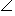1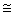2,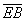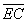Prove: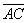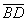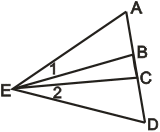4.

State whether or not each of the following triangle pairs is congruent. If so, state a reason.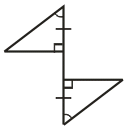5.

What does it mean for two triangles to be congruent?

6.

Does HL imply that SSA can be used to prove triangle congruent? Explain your answer.

7.

Does AAA guarantee that two triangles are congruent? Why or why not?

8.

What happens if the triangles share a side, part of a side, or part of an angle?

9.

State whether or not each of the following triangle pairs is congruent. If so, state a reason.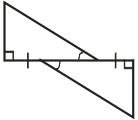10.

Given:12,34,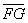Prove:CE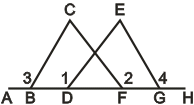Congruent Triangles
edHelper subscribers - Create a new printable

Number of Keys
 Select the number of different printables: 1 key 2 keys 3 keys 4 keys 5 keys

Number of Pages (for each key)
 Select the number of pages: 1 page 2 pages 3 pages 4 pages